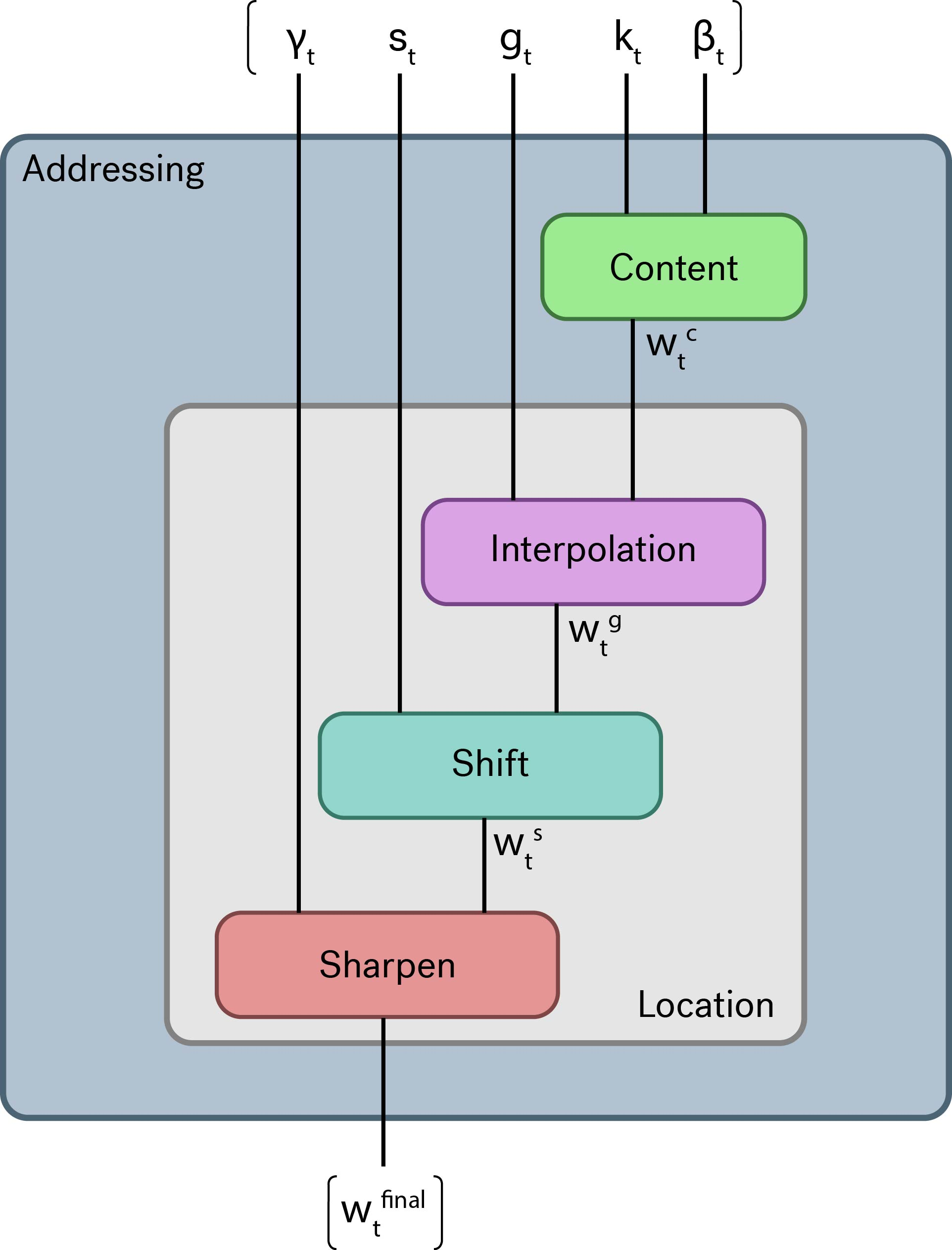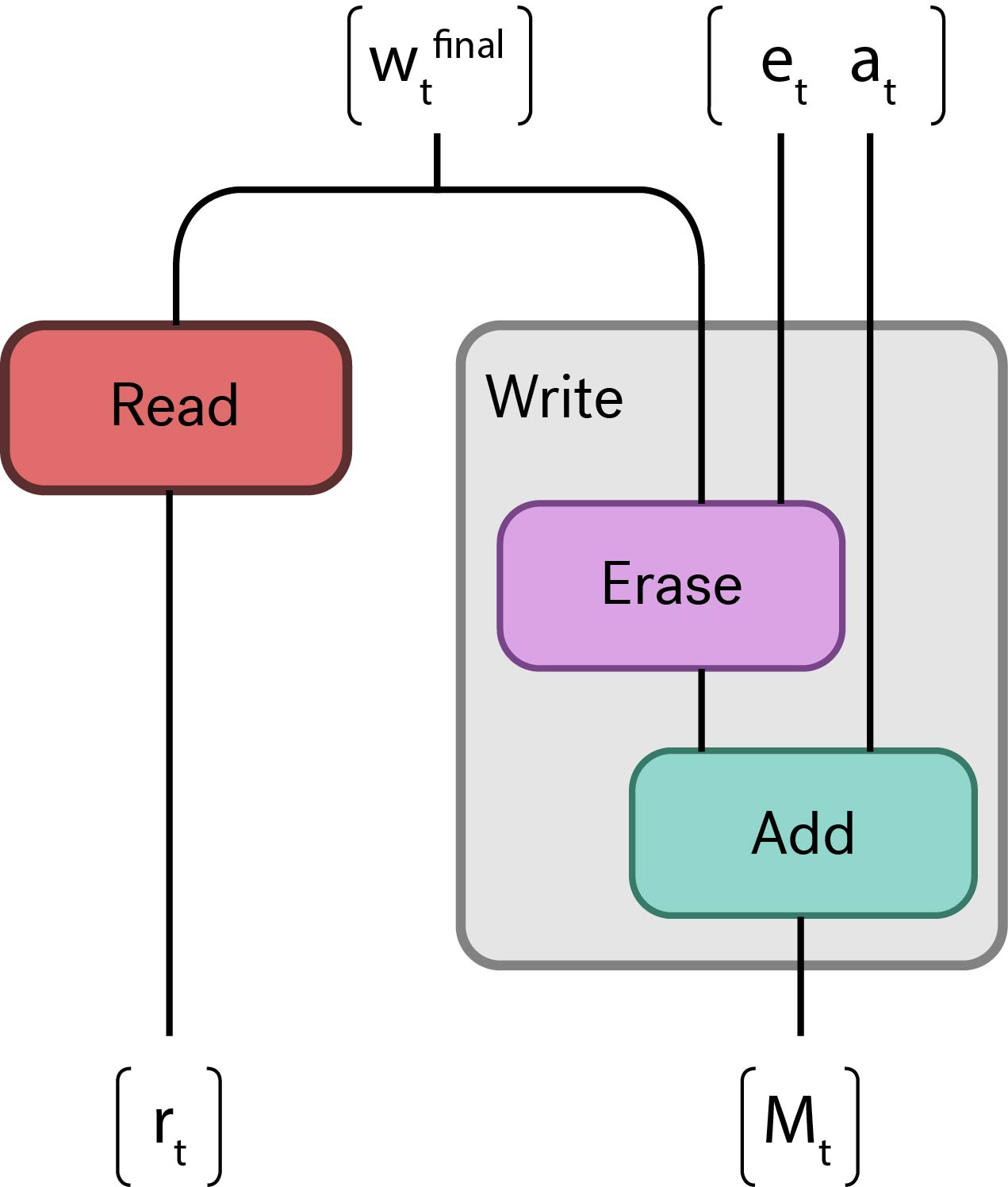# The Neural Turing Machine

This article serves to briefly outline the design of the Neural Turing Machine (NTM), a backpropogatable architecture that can (among many possibilities) learn to dynamically execute programs.

The original paper.

I’ve added some specifications about the NTM’s architecture that the paper excludes for the sake of generality. These will be discussed upon presentation.

The Neural Turing Machine was proposed by Graves et al. as a Turing-Complete network capable of learning (rather complex) programs. Inspired by the sequential nature of the brain, and the large, addressable memory of the computer.

##### The NTM is composed of five modules:
• The controller
• The write module
• The memory# The Controller

The controller acts as the interface between input data and memory. It learns to manage its own memory through addressing.

The paper maintains that the controller can be of any format, it simply needs to read in data and produce the outputs required by the sub-modules. They choose an LSTM for their implementation.

##### The parameters depended upon by the sub-modules are:
• $\mathbf{k_{t}}$ the key vector; Compared against the memory when addressing by content similarity.
• $\beta_{t}$ the key strength; A weighting effecting the precision of the content addressing.
• $g_{t} \in (0,1)$ the blending factor; A weight to blend between content addressing and previous time-step addressing.
• $\mathbf{s_{t}}$ the shift weighting; A normal distribution across the allowed shift amounts.
• $\gamma$ the sharpening exponent; Serves to sharpen the final address
• $\mathbf{e_{t}} \in (0,1)^{N}$ erase vector; Similar to an LSTM, decides what memory from the previous time-step to erase.
• $\mathbf{a_{t}}$ add vector; The data to be added to memory.This module generates a window over the memory for the read and write heads.

## Accessing by content similarity

This module effectively laps over the memory comparing each block to the key vector $\mathbf{k_{t}}$ and creates a normally distributed weighting over the memory based of the similarity.

$w^{c}_{t}(i) = softmax(\beta_{t}\delta(K_{t}, M_{t}(i)))$ where $\delta(a, b) = \dfrac{a \cdot b}{\vert a \vert \cdot \vert b \vert}$ is an example difference function.

## Accessing by location

This module has 3 steps:

1. The controller decides how much of the previous time-step’s weighting $\mathbf{w}^{final}_{t-1}$ should be preserved (using $g_{t}$).
2. It then performs a shift of the weighting (using $\mathbf{s}_{t}$).
3. Finally it sharpens (using $\gamma_{t}$) and normalizes the weighting.Pretty straight-forward.

## Writing

Writing is performed in two steps, similar to how an LSTM updates its state.

1. The erase vector $\mathbf{e_{t}}$ removes memory that is no longer relevant.
2. The new data is placed in memory (using $\mathbf{a_{t}}$).

# Significance

This architecture has already had an impact on a multitude of research projects (notably, the Neural GPU) and I have great faith it will continue to do so. There’s been much discussion over the past couple of years about computers programming themselves, and I believe this has been the greatest stride towards that end-goal.

The Neural Turing Machine is a reactionary computer, changing behaviour based on its “environment”. It certainly will play a major role in setting precedence for the way neural networks will be applied for the purpose of AI.

I look forward to reading more of the work done by the Google DeepMind and OpenAI teams.

If there are any errors in my description please do not hesistate to reach out to me at hello@aidangomez.ca.